# Dimensioning Tools

The dimensioning tools in Slide2 allow you to easily measure and display lengths and angles anywhere on the model, as shown in the following figure.

Length, width, height and angle dimensions added to slope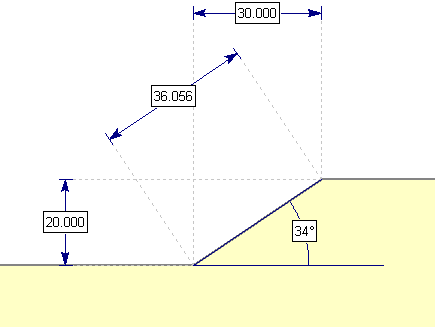Dimension Length, X, Y

To dimension the length, X distance, or Y distance between any two points in the view:

1. Select Dimension Length, Dimension X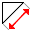or Dimension Y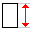from the toolbar or the Add Tool sub-menu of the Tools menu.
2. Select two points between which you would like to measure the distance. You can enter the points graphically with the mouse, or enter x,y coordinates in the prompt line.
3. TIP: The Snap options allow you to easily snap exactly to vertices, boundaries, or extension lines of vertices or boundaries.

4. As you move the mouse, the dimension (length, X distance or Y distance) between the two points will be displayed. When you are satisfied with the location of the dimension tool, click the mouse and it will be added to the view.

Dimension Angle

To dimension an angle anywhere on the model:

1. Select Dimension Angle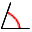from the toolbar or the Add Tool sub-menu of the Tools menu.
2. Enter two points defining a reference direction, from which you would like to measure an angle.
3. As you move the mouse, the angle formed between the first two points and the current cursor location (third point) will be displayed. When the desired angular measurement is displayed, click the mouse and the angle dimension tool will be added to the view.

Dimension Ratio

To dimension a ratio of a slope:

1. Select Dimension Ratio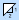from the toolbar or the Add Tool sub-menu of the Tools menu.
2. Enter two points defining a reference direction, from which you would like to measure a ratio.
3. When the desired angular measurement is displayed, click the mouse and the angle dimension tool will be added to the view.

TIP: After a dimension tool has been added, it can be moved, re-sized, formatted, etc. For example, you can specify the number of decimal places, or even replace the dimension value with customized text. See the Edit Tools Overview topic for details.